# 1st PUC Physics Question Bank Chapter 3 Motion in a Straight Line

## Karnataka 1st PUC Physics Question Bank Chapter 3 Motion in a Straight Line

### 1st PUC Physics Motion in a Straight Line TextBook Questions and Answers

Question 1.
In which of the following examples of motion, can the body be considered approximately a point object:

1. a railway carriage moving without jerks between two stations.
2. a monkey sitting on top of a man cycling smoothly on a circular track.
3. a spinning cricket ball that turns sharply on hitting the ground.
4. a tumbling beaker that has slipped off the edge of a table.

1. The railway carriage moving without jerks between two stations, so the distance between two stations is considered to be large as compared to the size of the train. Therefore the train is considered as a point object.
2. As the distance covered by the monkey is large in a reasonable time, so the monkey is considered a point object.
3. A point object does not have a spinning motion. Therefore ball can not be considered a point object.
4. As the beaker is tumbling and then slips off, so the distance covered by it is not large in a reasonable time. Therefore it is not treated as a point object.

Question 2.
The position-time (x-i) graphs for two children A and B returning from their school! O to their homes P and Q respectively are shown in Fig. 3.19. Choose the correct entries in the brackets below;

1. (A/B) lives closer to the school than (B/A)
2. (A/B) starts from the school earlier than (B/A)
3. (A/B) walks faster than (B/A)
4. A and B reach home at the (same/ different) time.
5. (A/B) overtakes (B/A) on the road (once/twice).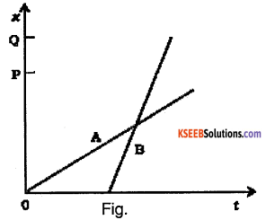1. The distance between P and O (the school) is less than the distance between Q and O. (from the graph)
⇒ A lives are closer to the school than B.
2. From the graph, we see that the line for A starts before the line for B.
⇒ A starts from school earlier than B.
3. Velocity = Slope of the x-t graph. Clearly, the slope for B > slope for A.
⇒ B walks faster than A
4. From the graph, we see that both lines end at the same time.
⇒ A and B reach home at the same time.
5. From the graph, we see that the lines intersect once. Also, B walks faster.
⇒ B overtakes A on the road once.Question 3.
A woman starts from her home at 9.00 am, walks at a speed of 5 km h-1 on a straight road up to her office 2.5 km away, stays at the office up to 5.00 pm, and returns home by an auto with a speed of 25 km h-1. Choose suitable scales and plot the x-t graph of her motion.
Time for the woman to reach her office = $$\frac{2.5 \mathrm{km}}{5 \mathrm{km} \mathrm{h}^{-1}}$$ = 30 min.
∴ She reaches her office at 9.30 am.
Time for the woman to return home = $$\frac{2.5 \mathrm{km}}{25 \mathrm{km} \mathrm{h}^{-1}}$$ = 0.1 h = 6 min.
∴ She reaches her home at 5.06 pm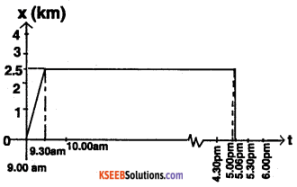Question 4.
A drunkard walking in a narrow lane takes 5 steps forward and 3 steps backward, followed again by 5 steps forward and 3 steps backward, and so on. Each step is 1 m long and requires 1 s. Plot the x – t graph of his motion. Determine graphically and otherwise how long the drunkard takes to fall in a pit 13 m away from the start.From the above table, see that every 8 s the drunkard moves 2 m forward.By looking at the shape of the graph, we can see that the graph for the entire motion will be like this:-From the graph, we see that the drunkard will fall into the pit at 137 s.
Analytically:-
The drunkard covers 2 m every 8 s.
∴ He will cover 8 m in 32 s. When he takes 5 steps forwards, he will fall into the pit.
∴ Total time = 32 + 5 = 37 s.

Question 5.
A jet airplane travelling at the speed of 500 km h-1 ejects its products of combustion at the speed of 1500 km h-1 relative to the jet plane. What is the speed of the latter with respect to an observer on the ground?
Velocity of jet =υJ = + 500 km h-1 (away from observer)
Relative velocity of ejection with respect to jet = υej = – 1500 kmh-1.
If υis the velocity of ejected products, then =Ve-Vj
=>  ve =ve. + vi = – 1500 + (500) = – 1000 km h-1
∴ veg = ve-vg = – 1000 km h_1  (ug=0)

Question 6.
A car moving along a straight highway with a speed of 126 km h-1 is brought to a stop within a distance of 200 m. What is the retardation of the car (assumed uniform), and how long does it take for the car to stop?
Answer: v0 = 126 km h-1
= 126 × $$\frac{5}{18}$$ m s-1
= 35 m s-1
Stopping distance = (x – x0) = 200 m
Since the car is stopped , v = 0.
v2 = v02 + 2 a (x – x0)
0 = 35² + 2a (200)
⇒ a = – 3.06 m s-2
Retardation = 3.06 m s-2
v = v0 + at
⇒ 0 = 35 – 3.06 t
⇒ t = 11.4 s
Time for car to stop = 11.4 s.Question 7.
Two trains A and B of length 400 m each are moving on two parallel tracks with a uniform speed of 72 km h-1 in the same direction, with A ahead of B. The driver of B decides to overtake A and accelerates by 1 m s-2. If after 50 s, the guard of B Just brushes past the driver of A, what was the original distance between them?
Initially,Relative Acceleration a = 1 ms-2
v0 =72 km h-1 = 72 × $$\frac{5}{18}$$ m s-1
= 20 ms-1
Relative displacement = x
Relative velocity initially = V0 – V0 = 0
X = 1/2 at²
= 1/2 × 1 × 50² = 1250 m
Original distance between the trains = 1250 m.

Question 8.
On a two-lane road, car A Is travelling at a speed of 36 km h-1. Two cars B and C approach car A in opposite directions with a speed of 54 km h-1 each. At a certain instant, when the distance AB is equal to AC, both being 1 km, B decides to overtake A before C does. What minimum acceleration of car B is required to avoid an accident?VA= 36 km h-1 = 10 m s-1
VB = Vc = 54 km h-1 = 15 m s-1
Velocity Of B with respect to A
= VBA = VB – VA
= 15m -10 = 5 m s-1
Velocity of C with respect to A .
VCA = VC – VA
= 15 – ( -10) = 25 m s-1
S = VCA t
1000 m = 25 × t
⇒ t = 40 s
C will take 40 s to cover AC. In that time, B must cover AB to overtake and avoid collisions.
s = VBA t + 1/2 at²
1000 = 5 × 40 + 1/2 × a × 40²
⇒ a = 1 ms-2
Acceleration of B = 1 ms-2

Question 9.
Two towns A and B are connected by regular bus service with a bus leaving In either direction every T minutes. A man cycling with a speed of 20 km h-1 In the direction A to B notices that a bus goes past him every 18 min in the direction of his motion, and every 6 min in the opposite direction. What is the period of the bus service and with what speed (assumed constant) do the buses ply on the road?
Let the speed of the bus be v km h-1
Distance covered by one bus before the next one leaves the same town is vT For buses going in the same direction as the cyclist, frequency of the bus is 18 min i.e.,
$$\frac{v T}{\text { relative velocity of the bus w.r.t. the cyclist }}$$ = 18 min
$$\frac{v T}{v-20}$$ = 18 ……………… (1)
Similarly, for buses going in the opposite direction, frequency of the bus = 6 min
$$\frac{v T}{\text { relative velocity of the bus w.r.t. the cyclist }}$$ = 6 min
$$\frac{v T}{v+20}$$ = 6 ……………… (2)
$$(1) \div(2)$$
⇒ $$\frac{v+20}{v-20}$$
Substitute for v in (1)
$$\frac{40 \times T}{40-20}$$ =18 ⇒ T = 9 min.

Question 10.
A player throws a ball upwards with an initial speed of 29.4 ms-1.

1. What is the direction of acceleration during the upward motion of the ball?
2. What are the velocity and acceleration of the ball at the highest point of its motion?
3. Choose the X = 0 m and t = 0 s to be the location and time of the ball at its highest point, vertically downward direction to be the positive direction of X-axis, and give the signs of position, velocity, and acceleration of the ball during its upward, and downward motion.
4. To what height does the ball rise and after how long does the ball return to the player’s hands? (Take g = 9.8 m s-2 and neglect air resistance).

1. The direction of acceleration is vertically downwards (towards the Earth)
2. At the highest point, velocity = 0 m s-1 acceleration = g = 9.8 m s-2 vertically downwards.
3. During upward motion:

• position – positive
• velocity – negative
• acceleration – positive

During downward motion:

• position – positive
• velocity – positive
• acceleration – positive

4. v = 0 at the highest point
v0 = 29.4 m s-1
g = -9.8 m s-2
v² = v02 + 2 g h
0 = 29.4² = 2 × (-9.8) × 4
⇒ h = 44.1m
Height of ascension = 44.1 m
v = v0 + g t
0 = 29.4 – 9.8 t
t = 3 s
Time of ascension = Time of descension
∴ Time for the ball to return = 6 sQuestion 11.
Read each statement below carefully and state with reasons and examples, if it is true or false; A particle in one-dimensional motion

1. with zero speed at an instant may have non-zero acceleration at that instant
2. with zero speed may have non-zero velocity,
3. with constant speed must have zero acceleration,
4. with a positive value of acceleration must be speeding up.

1. True. A particle thrown upward has zero speed at the highest point but a = g = 9.8 ms_2  in a vertically downward direction.
2. False. Because the magnitude of velocity = speed. When speed is zero, velocity may not be non-zero.
3. True. Because the particle rebounds instantly with the same speed, it means it has infinite acceleration which is not possible.
4. False. It can be true only when the chosen positive direction is along the direction of motion.

Question 12.
A ball is dropped from a height of 90 m on a floor. At each collision with the floor, the ball loses one-tenth of its speed. Plot the speed-time graph of its motion between t = 0 to 12 s.
Velocity of the ball when it hits the ground = v
v0 = 0, h = 90 m, g = 9.8m s-2
v² = v02 + 2 g h
v² = 02 + 2 × 9.8 × 90
⇒ v = 42 ms-1
Time for descent = t
v = v0 + g t
42 = 0 + 9.8 t
t = 4.3 s
During the rebound, the initial speed of the ball = 9/10 of v
= 9/10 × 42
= 37.8 ms-1
Total time for ascent and descent = t1
Net displacement = 0
0 = 37.8 t – 1/2 gt²
37.8 t = 1/2 × 9.8 × t² ⇒ t = 7.7 s
Time at which maximum height is achieved = 4.3 + 1/2 7.7
= 8.15 s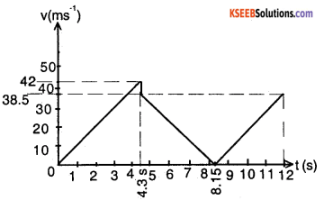Question 13.
Explain clearly, with examples, the distinction between:
(a) magnitude of displacement (sometimes called distance) over an interval of time, and the total length of path covered by a particle over the same interval;

(b) magnitude of average velocity over an interval of time, and the average speed over the same interval. [Average speed of a particle over an interval of time is defined as the total path length divided by the time interval]. Show In both (a) and (b) that the second quantity is either greater than or equal to the first. When is the equality sign true? [For simplicity, consider one-dimensional motion only].
(a) Consider a person who leaves home for work in the morning and returns home in the evening.
The magnitude of the displacement of the person during the interval is zero but the total length of path covered = 2 × distance between the home and the workplace.
Magnitude of displacement is the magnitude of the shortest length between the two points. The second quantity is clearly always greater than or equal to the first quantity. The equality holds if the body has not moved at all (path length = magnitude of displacement = 0)

(b) Average velocity = $$\frac{\Delta x}{\Delta t}$$. If Δ X is zero (like in the example explained in (a)) then average velocity = 0.
Average speed = $$\frac{\text { Total path length }}{\text { Time taken }}$$
2 × $$\frac{\text { Distance between the home and the work place }}{\text { Time taken to traverse this distance }}$$
= Clearly average speed ≥ magnitude of average velocity. Equality exists when body does not undergo any motion.

Question 14.
A man walks on a straight road from his home to a market 2.5 km away with a speed of 5 km h-1. Finding the market closed, he instantly turns and walks back home with a speed of 7.5 km h-1. What is the

1. magnitude of average velocity, and
2. average speed of the man over the interval of time

(i) 0 to 30 min,
(ii) 0 to 50 min,
(iii) 0 tp 40 min?
[Note: You will appreciate from this exercise why it is better to define average speed as total path length divided by time, and not as the magnitude of average velocity. You would not like to tell the tired man on his return home that his average speed was zero !]
v1 = 5 km h-1, v2 = 7.5 km h-1, d = 2.5 km
Time for the man to reach the market
= t1 = d/v1= 2.5/5 = 0.5 h = 30 min
Time for the man to return from the market
= t2= d/v2 = 2.5/7.5 = 1/3 h= 20 min

1. To find the average velocity,
Average velocity = $$\frac{\text { Total path length }}{\text { Total time taken }}$$

• During the interval between 0 to 30 min,
Displacement = 2.5 km
time = 30 minutes
∴ Average velocity = $$\frac{2.5 \mathrm{km}}{30 \mathrm{min}}$$ = 5 km h-1
• interval between 0 and 50 minutes,
Displacement = 0
∴ Average velocity = 0
• During between 0 to 40 minutes,
Displacement = 2.5 km – 7.5 kmh-1 × 10 min = 1.25 km
time = 40 minutes
∴ Average velocity = $$\frac{1.25 \mathrm{km}}{40 \mathrm{min}}$$ = 1.875 km h-1

2. To find the average speed:
Average speed = $$\frac{\text { Total path length }}{\text { Total time taken }}$$

• During the interval between 0 to 30 min
Path length = 2.5 km
time = 30 minutes
∴ Average speed = $$\frac{2.5 \mathrm{km}}{30 \mathrm{min}}$$ = 5 km h-1
• During the interval between 0 and 50 minutes,
Path length = 2.5 km + 2.5 km
Time taken = 50 minutes
∴ Average speed = $$\frac{2.5 \mathrm{km}+2.5 \mathrm{km}}{50 \mathrm{min}}$$ = 6 km h-1
• During 0 to 40 minutes
Path length = 5 kmh-1 × 30 min + 7.5 kmh-1 × 10 min
2.5 km + 1.25 km = 3.75 km
time = 40 minutes
∴ Average velocity = $$\frac{3.75 \mathrm{km}}{40 \mathrm{min}}$$ = 5.625 km h-1Question 15.
In Exercises 3.13 and 3.14, we have carefully distinguished between average speed and magnitude of average velocity. No such distinction is necessary when we consider the instantaneous speed and the magnitude of velocity. The instantaneous speed is always equal to the magnitude of instantaneous velocity. Why?
When we consider arbitrarily small intervals of time, the magnitude of displacement is always equal to the magnitude of distance.

Question 16.
Look at the graphs (a) to (d) (Fig. 3.20) carefully and state, with reasons, which of these cannot possibly represent the one-dimensional motion of a particle.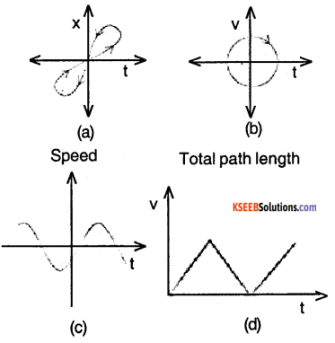None of the four graphs can represent the one-dimensional motion of the particle.
(a) A particle cannot have two different positions at the same time.
(b) A particle cannot have two values of velocity at the same time.
(c) Speed is always positive.
(d) Total path length of a particle can never decrease with time.

Question 17.
Figure 3.21 shows the X- t plot of the one-dimensional motion of a particle. Is it correct to say from the graph that the particle moves in a straight line for t < 0 and on a parabolic path for t > 0? If not, suggest a suitable physical context for this graph.No, it is wrong to make such statements about the trajectory of the particle because an x-t graph does not show the particle’s trajectory.
Context:
Consider a body dropped from the top of a tower at time t = 0. If the vertically downward direction is chosen as the positive direction, then the body’s x -t graph would resemble the one given in the question.

Question 18.
A police van moving on a highway with a speed of 30 km h-1 fires a bullet at a thief’s car speeding away In the same direction with a speed of 192 km h-1. If the muzzle speed of the bullet is 150 m s-1, with what speed does the bullet hit the thief’s car?
Speed of the police van = vp = 30 km h-1 = 25/3 ms-1.
Speed of the thief’s car is vt = 192 km h-1 = 160/3 ms-1
Speed of the bullet = vb = 150 ms-1
Speed of the bullet with respect to the police car = vb + vp = 150 + 25/3 = 475/3 msv-1
Speed with which the bullet hits the thief’s car = Speed of the bullet with respect to the thief’s car
= 475/3 – vt
= 475/3 – 160/3
=105 ms-1

Question 19.
Suggest a suitable physical situation for each of the following graphs (Fig 3.22):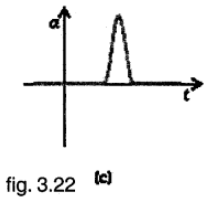(a) The x-t graph shows that initially x = 0, attains a certain value of x, again x becomes zero, and then x increases in the opposite direction till it settles at a constant x (i.e., comes to rest). Therefore, it may represent a physical situation such as a ball (initially at rest) on being hit moves with constant speed, rebounds from the wall with less rebound speed, and then moves to the opposite wall and then stops.

(b) From the υ-t graph, it follows that velocity changes sign again and again with the passage of time and every time losing some speed. Therefore, it may represent a physical situation such as a ball falling freely (after throwing up), on striking the ground rebounds with reduced speed after each hit against the ground.

(c) The a-t graph shows that the body gets accelerated for a short duration only. Therefore, it may represent a physical situation such as a ball moving with uniform speed is hit with a bat for a very small time interval.

Question 20.
Figure 3.23 gives the x-t plot of a particle executing one-dimensional simple harmonic motion. Give the signs of position, velocity and acceleration variables of the particle at t= 0.3 s, 1.2 s, -1.2 s.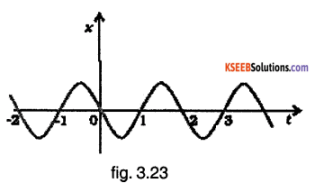Let the maximum amplitude of the sine wave be A, where A is positive. We can see that the particle obeys the
equation x = – A sin $$\left(\frac{2 \pi \mathrm{t}}{\mathrm{T}}\right)$$
Where T = 2 s = period of the sine wave
∴ position = x = – A sin (π t)
velocity – v = $$\frac{dx}{dt}$$ = – Aπ cos (π t)
acceleration = a = $$\frac{dv}{dt}$$ = Aπ² sin(π t)
At t = 0.3 s
x = – A sin (0.3π) = negative
v = – A cos (0.3π) = negative
a = A π² sin (0.3π) = positive
Since sin(0.3π) > 0 and cos(0.3π) > 0
At t = 1.2 s
x = – A sin (1.2π) = – A sin (1.2π) = positive
v = – A π cos (1.2π) = – A cos (1.2π) = positive
a = Aπ² sin (1.2π) = negative
since sin(1.2π)<0 and cos(1.2π) < 0
At t = – 1.2 s
x = – A sin (- 1.2π) = A sin (1.2π)= negative
v = – A it cos(- 1.2π) = – Aπ cos (1.2π) = positive.
a = Aπ² sin (- 1.2π) = – Aπ 2sin(1.2π) = positive
Since sin(-θ) = – sinθt cos(-θ) = cosθ sin(1.2π) <0 and cos(1.2π) < 0

Question 21.
Figure 3.24 gives the x-t plot of a particle in one-dimensional motion. Three different equal intervals of time are shown. In which interval is the average speed greatest, and in which is it the least? Give the sign of average velocity for each Interval.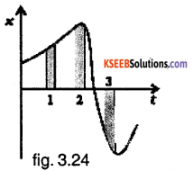The average speed of particle = slope of x-t graph. The slope of x -t is maximum at t = 3, and minimum at t = 2. Therefore, the average speed of a particle is greatest at t = 3 add least at t = 2. o > 0 in 1 and 2, and υ< 0 in 3.

Question 22.
Figure 3.25 gives a speed-time graph of a particle In motion along a constant direction. Three equal intervals of time are shown. In which interval is the average acceleration greatest in magnitude? In which interval is the average speed greatest? Choosing the positive direction as the constant direction of motion gives the signs of v and an in the three Intervals. What are the accelerations at points A, B, C, and D?The magnitude of the slope of the speed-time graph is greatest for interval 2. Hence average acceleration is greatest in magnitude in interval 2. The value of speeds in interval 3 is the highest. Hence the average speed in interval 3 is the highest. Sign of a = sign of the slope of the speed-time graph, a > 0 for 1 and 3 a < 0 for 2. At points, A, B, C, and D, the tangent to the curve will be parallel to the time axis. Hence slope at the points is zero,
∴ a = 0 at points A, B, C, and D.Question 23.
A three-wheeler starts from rest, accelerates uniformly with 1 m s-2 on a straight road for 10 s, and then moves with uniform velocity. Plot the distance covered by the vehicle during the nth second (n = 1, 2, 3….) versus n. What do you expect this plot to be during accelerated motion: a straight line or a parabola?
Initial velocity u = 0.
Acceleration a = 1 ms-2.
Acceleration time = 10 s
Let sn be the total displacement (or distance in this case) in time ‘n’ seconds.
sn = ut + 1/2 at²
sn = 0(n) + 1/2 a(n²) = 1/2 an² for n ≤ 10
Distance covered in ‘n’th second dn = sn – sn – 1
= 1/2 an² – 1/2 a(n-1)²
= an – 1/2 a (for n ≤ 10)
= (n – 1/2) m
At the end of 10 seconds, velocity acquired v = u + at = 0 + a (10)
10 a = 10 ms-1
Distance covered in the ‘n’th second = 10m for n >10
∴ dn = {(n-1/2)m n ≤ 10,
10m n > 10
The plot is a straight line inclined to the time axis for uniformly accelerated motion. (It is a straight line parallel to the time axis for uniform motion).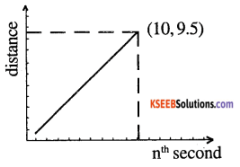Question 24.
A boy standing on a stationary lift (open from above) throws a ball upwards with the maximum initial speed he can, equal to 49 m s-1. How much time does the bail take to return to his hands? if the lift starts moving up with a uniform speed of 5 m s-1 and the boy again throws the ball up with the maximum speed he can, how long does the ball take to return to his hands?
Initial velocity n = 49 ms-1
Acceleration g = -9.8 ms²
Displacement s = 0
s = ut + 1/2 at²
0 = 49 t + 1/2 (-9.8) t²
⇒ t = 0 or t = 10 s
t = 0 represents initial time. The required ‘t’ here is t = 10 s. In the case where the lift also moves, the relative velocity of the ball with respect to the boy remains the same, i.e, 49 ms-1. The relative displacement is also 0. Hence the time required in this case is also 10 s.

Question 25.
On a long horizontally moving belt (Fig. 3.26), a child runs to and fro with a speed 9 km h-1 (with respect to the belt) between his father and mother located 50 m apart on the moving belt. The belt moves with a speed of 4 km h-1. For an observer on a stationary platform outside, what is the

1. speed of the child running in the direction of motion of the belt?.
2. speed of the child running opposite to the direction of motion of the belt?
3. time taken by the child in (1) and (2)?

Which of the answers alter If motion is viewed by one of the parents?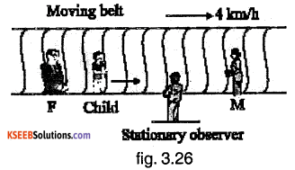1. Speed of the child running in the direction of motion of the belt = Speed of the child with respect to the belt + Speed of the belt with respect to the ground
= 9 + 4 = 13 km h-1.
2. Speed of the child running opposite to the direction of motion of the belt = speed of the child with respect to the belt speed of the belt with respect to the ground
= 9 – 4 = 5 km h-1.
3. Time taken by the child in both cases is the same because the speed of the child with respect to the belt does not change.
Speed v = 9 km h-1 = 2.5 ms-1
Distance d = 50 m
Time t = $$\frac{\text { Distance }}{\text { Speed }}$$ = $$\frac{50}{2.5}$$ = 20 s
The answer to (a) and (b) when the motion is viewed by one of the parents is 9 km h-1. This is because the parents are also on the belt and are moving with respect to the ground. They only see the motion of the child with respect to the belt. In (c), the answer remains unaltered because the speed of the child with respect to the belt does not depend on the speed of the parents.

Question 26.
Two stones are thrown up simultaneously from the edge of a cliff 200 m high with Initial speeds of 15 m s-1 and 30 m s-1. Verify that the graph shown in Fig. 3.27 correctly represents the time variation of the relative position of the second stone with respect to the first. Neglect air resistance and assume that the stones do not rebound after hitting the ground. Take
g = 10 ms-2. Give the equations for the linear and curved parts of the plot.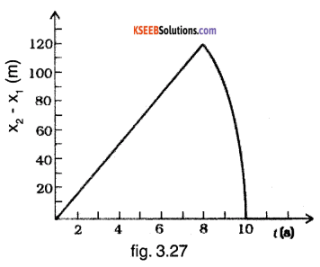Initial velocity of stone 1 is u1 = 15 ms-1
Initial velocity of stone 2 = u2 = 30 ms-1
Acceleration = g = -10ms-2 (vertically upwards direction is chosen as positive)
x1 = 200 + u1t + 1/2 at²
= 200 + 15 t – 5 t²
x2 = 200 + u2t + 1/2 at²
= 200 + 30 t – 5 t².
Also x1 = 0 for t = 8 s and
x2 = 0 for t = 10s.
∴ For 0 ≤ t ≤ 8 s
x2 – x1 = 15 t
At t = 8 s,
x2 – x1 = 120 m
For 8 < t; t ≤ 10 s
x2 – x1 = 200 + 30 t – 5 t² – 0
= 200 + 30 t – 5 t².
For t > 10 s,
x2 – x1 = 0
∴ The given graph is correct and because it matches with the equations obtained.Question 27.
The speed-time graph of a particle moving along a fixed direction is shown In Fig. 3.28. Obtain the distance traversed by the particle between
(a) t = 0 s to 10 s.
(b) t = 2 s to 6 s.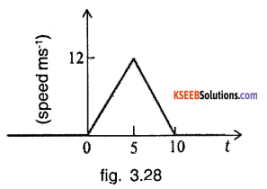What is the average speed of the particle over the intervals in (a) and (b)?.
(a) Distance covered = Area under the speed – time graph
= 1/2 × 10 × 12 = 60 m
Average speed = $$\frac{\text { Distance covered }}{\text { Time taken }}$$
= $$\frac{60 m}{10 s}$$ = 6 ms-1

(b) For 0 ≤ t ≤ 5 s,
Speed v = $$\frac{12}{5}$$ t ms-1
For 5 < t ≤ 10 s,
Speed v = 12 – $$\frac{12}{5}$$ (t – 5) ms-1
∴ Speed at t = 2 s is 4.8 ms-1 and speed at t = 6 s is 9.6 ms-1
Distance covered = Area of trapezium ABEF + Area of trapezium BCDE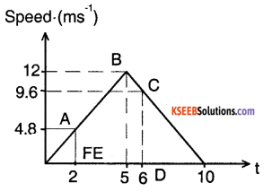= 1/2 (4.8 + 12) × 3 + 1/2 (12 + 9.6) × 1
= 36 m
Average speed = $$\frac{\text { Distance covered }}{\text { Time taken }}$$
= $$\frac{36}{6-2}$$ = 9 ms-1

Question 28.
The velocity-time graph of a particle in one-dimensional motion is shown in Fig. 3.29:Which of the following formulae are correct for describing the motion of the particle over the time-interval t1 to t2

1. x(t2) = x(t1 + v (t1) (t2 – t1 + (1/2) a (t2– t1
2. v(t2) = v(t1) + a(t2 – t1)
3. vaverage = (x(t2) – x(t1)/(t2-t1)
4. average = (v(t2 – v(t1))/(t2 – t1)
5. x(t2) = x(t1) + vaverage (t2 – t1) + (1/2) aaverage (t2 – t1
6. x(t2) – x(t1) = area under the v-t curve bounded by the t-axis and the dotted line shown.

1. Wrong, because it is not known whether the acceleration ‘a’ is constant.
2. Wrong, because it is not known whether the acceleration ‘a’ is constant.
3. Correct. By definition.
4. Correct. By definition
5. Wrong. The formula should contain v1 (t) instead of vaverage.
6. Correct By definition.

### 1st PUC Physics Motion in a Straight Line One Mark Questions and Answers

Question 1.
When do you say that a body is in motion?
A body is said to be in motion when it changes its position with respect to time and surroundings.

Question 2.
What is a particle?
A particle is a geometrical mass point.

Question 3.
Define displacement.
The change of position of a body in a particular direction is called displacement.

Question 4.
Can the displacement of a moving body be zero?
Yes

Question 5.
Define speed.
The rate of change of position of a body in any direction is known as speed.

Question 6.
Define velocity.
The rate of change of position of a body in a particular direction is called velocity or the Rate of displacement of a body is called velocity.Question 7.
Define uniform velocity.
If a body covers equal distances in equal intervals of time in a given direction, however small the intervals maybe then its velocity is said to be uniform.

Question 8.
Define variable velocity.
If the velocity of a body either changes in magnitude or in direction or both, then its velocity is said to be variable.

Question 9.
Define average velocity.
The average velocity of a body is defined as the ratio of its total displacement to the total time.

Question 10.
Define acceleration.
Rate of change of velocity of a body is called acceleration.

Question 11.
Define uniform acceleration.
If the velocity of a body changes by an equal amount in equal interval of time, however, small the interval maybe then its acceleration is said to be uniform.

Question 12.
What is a position-time graph?
A graph drawn by taking time along the x-axis and displacement along the y-axis is called a position-time graph.

Question 13.
What does the slope of the position-time graph indicate?
The slope of the position-time graph indicates velocity.

Question 14.
Draw the position-time graph of a particle which is at rest.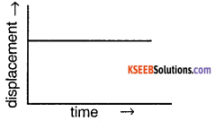Question 15.
Draw the position-time graph of an object starting from rest and moving with uniform velocity.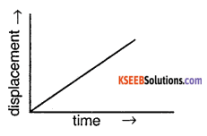Question 16.
What is a velocity-time graph?
A graph drawn by taking time along x-axis and velocity along the y-axis is called a velocity-time graph.

Question 17.
What does the slope of the velocity-time graph indicate?
The slope of the velocity-time graph indicates the acceleration.

Question 18.
What does the area under the velocity-time graph indicate?
Area under the velocity-time graph indicates the displacement.

Question 19.
An object is moving with uniform velocity. What Is its acceleration?
Zero

Question 20.
How do you determine the instantaneous velocity of a particle from the position-time graph?
Instantaneous velocity at any point is given by the slope of the tangent drawn to the position-time graph at that point.

Question 21.
Draw the velocity-time graph of a particle moving with

1. uniform velocity
2. variable velocity.

1)2)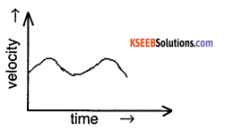Question 22.
Draw the velocity-time graph of an object starting from rest and moving with uniform acceleration.Question 23.
Draw the velocity-time graph of an object moving with constant retardation.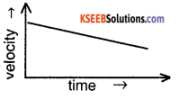Question 24.
Draw the position time of the particle which is initially at rest and moving with uniform acceleration.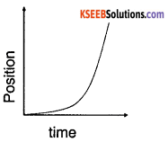Question 25.
What is the acceleration time graph?
A graph drawn by taking time along x-axis and acceleration along the y-axis is called a velocity-time graph.

Question 26.
Write the expression for the distance travelled by a body during the nth second of its motion.
Distance travelled by a body during the nth second of motion is given by
sn = u + a(n – 1/2)
where u is the initial velocity and a is the uniform acceleration.Question 27.
Write the expression for the acceleration in terms of distance travelled in two consecutive intervals of time.
Acceleration is given by,
a = $$\frac{s_{2}-s_{1}}{t^{2}}$$
where s1 and s2 are the distances travelled in two consecutive intervals of time ‘t’ seconds each.

Question 28.
What is relative velocity?
The velocity of a particle in motion relative to another particle is called relative velocity.

Question 29.
When is the relative velocity of two bodies maximum?
Relative velocity of two bodies is maximum when they are moving in opposite directions.

Question 30.
What does the area of a-t graph indicate?
Area of a-t graph represents the change in velocity of the body in a given time interval.

Question 31.
Draw the a-t graph of a body moving with uniform acceleration.Question 32.
Why is it not necessary for a body following another, to stop, to avoid collision?
If the relative velocity is zero, no collision will occur.

Question 33.
If in case df a motion, displacement is directly proportional to the square of the tune elapsed, what do you think about its acceleration, le, constant or variable? Explain why?
x ∝ t²
⇒ x = kt² Where k is a constant
a = $$\frac{d}{d t}\left(\frac{d x}{d t}\right)$$ = $$\frac{d}{d t}$$ (2 k t)
= 2k = constant
∴ Acceleration is constant.

Question 34.
Why does the earth Impact the same acceleration to all bodies?
Since acceleration is the force on unit mass, the acceleration due to gravity is constant.

Question 35.
What will be the nature of the velocity-time graph for a uniform motion?
The v -t graph will be a line parallel to the time axis.Question 36.
The position coordinate of a moving particle is given by x = 6 + 18t + 9t2(x is in metres and t is in seconds). What is its velocity at t s 2s?
x = 6 + 18 t + 9t²
v= $$\frac{\mathrm{d} x}{\mathrm{dt}}$$ = 18 + 18 t
v at t = 2 s = 18 + 18 (2)
= 54 ms-1

Question 37.
A ball is thrown straight up. What is its velocity and acceleration at the top?
At the top, v = 0, a = g.

### 1st PUC Physics Motion in a Straight Line Two Mark Questions and Answers

Question 1.
Distinguish between speed and velocity.

1. Speed is the rate of change of position of a body in any direction while velocity is the rate of change of position of a body in a particular direction.
2. Speed is a scalar quantity whereas velocity is a vector quantity.

Question 2.
What is a velocity-time graph? What Is Its importance?
A graph drawn by taking time along the x-axis and velocity along the y-axis is called a velocity-time graph. The velocity-time graph can be used

1. To find the nature of the motion of a body.
2. To determine the velocity of a body at any instant.
3. To calculate the displacement of a body in a given time.

Question 3.
If V1 and v2 are the velocities of two bodies then what is their relative velocity when they move in the

1. same and
2. opposite direction?

1. When the bodies move in the same direction, the relative velocity of 1st body w.r.t 2nd is v12 = v1 – v2
2. When the bodies move in the opposite directions, the relative velocity of 1st body w.r.t 2nd is v12 = v1 + v2.

Question 4.
A car moving with a uniform velocity of 54 kmph is brought to rest in travelling a distance of 5m What is the retardation produced by brake?
Initial velocity of the car.,
u = 54km/hr
= $$\frac{54 \times 1000}{3600}$$ = 15 ms-1
Final velocity of the car, v = 0.
Let a be the retardation of the car. Then using the relation.
v² = u² + 2as
0² = 15² + 2a × 5
a = $$-\frac{15 \times 15}{2 \times 5}$$ = $$\frac{225}{10}$$ = -22.5 ms-2.

Question 5.
An automobile moving with a uniform velocity of 500ms-1 is brought to rest in travelling a distance of 5m. What is the acceleration produced by the brakes?
Initial velocity of the car, u = 500ms-1
Final velocity of the car v = 0
Let a be the acceleration of the car.
Then using the relation.
v² = u² + 2aS
0² = 500² + 2a × 5
a = $$-\frac{500^{2}}{10}$$ = – 25 × 103mS-2.Question 6.
The equation of motion of a body is given by S = 2t + 2t², where S is in meter and time in second. What is the acceleration of the body?
S = 2t + 2t². This is of the standard form S = ut + 1/2 at²
By comparison, 1/2 a = 2 or a = 4m/s².

Question 7.
Draw a velocity-time graph for a particle in the following situations:

1. Starts from rest and moves with uniform acceleration
2. Moving with uniform retardation.

1. Body moving the uniform acceleration.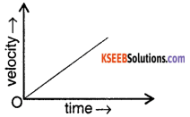2. Body moving with uniform retardation.Question 8.
Define velocity and acceleration.
Velocity is defined as the rate of displacement. Acceleration is known as the rate of change of velocity.

Question 9.
Two parallel nail tracks run North-South. Train A moves due north with a speed of 54 km h-1 and train B moves due south with a speed of 90 km h-1. What is the relative speed of B with respect to A in ms-1?
VA = 54 km h-1
VB = – 90 km h-1
(north direction is chosen as positive)
VBA = VB – VA
= – 90 – 54 = -144 km h-1
= – 40 ms-1

Question 10.
Differentiate between the average speed and the instantaneous speed of an object.
The distance covered in unit time is called average speed.
Vavg = $$\frac{x\left(t_{2}\right)-x\left(t_{1}\right)}{t_{2}-t_{1}}$$
The speed at any instant of time is called instantaneous speed.
Vinst= $$\Delta \mathrm{t} \rightarrow 0 \frac{\Delta \mathrm{x}}{\Delta \mathrm{t}}$$

Question 11.
The velocity-time graph of a moving object is shown below. What is the acceleration of the object? Also, draw a displacement time graph for the motion of the object.Acceleration = Zero (because velocity is constant) Displacement time graph is shown below: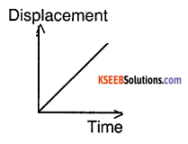Question 12.
Two straight lines are drawn on the same displacement time graph make angles 30° and 60° with the time axis respectively in the figure. Which line represents greater velocity? What is the ratio of the velocity of line A to that of line B?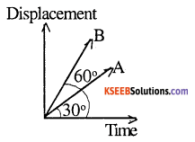Velocity = Slope of x -t graph
VA = tan 30° = $$1_{\sqrt{3}}$$
VB = tan 60° = Clearly, VB > VA.
$$\frac{V_{A}}{V_{B}}$$ = $$\frac{1_{\sqrt{3}}}{\sqrt{3}}$$ = $$\frac{1}{3}$$

### 1st PUC Physics Motion in a Straight Line Three Mark Questions and Answers

Question 1.
The distance x travelled by a body In a straight line is directly proportional to t². Decide on the type of motion associated. If x ∝ t3 what change will you observe?
x ∝ t² = kt² where k is a constant
a = $$\frac{d}{d t}\left(\frac{d x}{d t}\right)$$ = $$\frac{\mathrm{d}}{\mathrm{dt}}$$ (2 k t )
= 2 k
∴ Acceleration is constant / uniform.
If x ∝ t3 where k is a constant
a = $$\frac{d}{d t}\left(\frac{d x}{d t}\right)$$ = $$\frac{\mathrm{d}}{\mathrm{dt}}$$ (3 k t²)
= 6 k t
∴ Acceleration is non-uniform

Question 2.
Draw the following graph for an object under free-fall:

1. Variation of acceleration with respect to time.
2. Variation of velocity with respect to time
3. Variation of distance with respect to time.

1.2.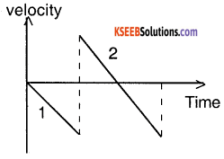Line 2 appears only when the body bounces.
3.Question 3.
A body that starts from rest accelerates uniformly along a straight line at the rate of 10 ms-2 for 5 seconds. It moves for 2 seconds with a uniform velocity of 50 ms-1. Then it retards uniformly and comes to rest in 3s. Draw the velocity-time graph of the body and find the total distance travelled by the body.Total distance travelled = Area under the v – t graph
= 1/2 × 50 × (10 + 2)
= 300 m

Question 4.
The displacement (in metre) of a particle moving along x-axis given by x = 18 t + 5t². Calculate:-

1. the instantaneous velocity at t = 2s
2. average velocity between ta 2 s and 3 s
3. instantaneous acceleration

x = 18 t + 5 t²
v(t) = $$\frac{d x}{d t}$$ = 18 + 10 t,
a(t) = $$\frac{d v}{d t}$$ = 10 ms-2
1. v (2) = 18 + 10 (2) = 38 ms-1
2. v (2) = 38 ms-1

v(3) = 18 + 10 (3) = 48 ms-1
Since acceleration is constant,
Vavg $$\frac{v(3)+v(2)}{2}$$ = $$\frac{48+38}{2}$$ = 43 ms-1.

3. Instantaneous acceleration a = 10 ms-2.

### 1st PUC Physics Motion in a Straight Line FourFive Mark Questions and Answers

Question 1.
Derive an expression for the velocity of the particle after time ‘t’ or Derive v = u + at using a v-t graph.
Consider a particle moving along a straight line with a constant acceleration ‘a’. Let u be its initial velocity and v be its velocity after a time t. The velocity-time graph AB of this particle is shown in the figure.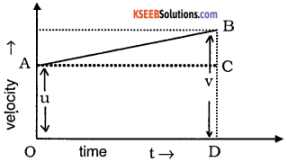Acceleration of the particle is given by the slope of the v-t graph.
From the graph,
slope of the line AB = $$\frac{B C}{A C}=\frac{B C}{O D}=\frac{B D-C D}{O D}$$
But, BD = v, the final velocity,
OA = u, the initial velocity,
OD = t, the time.
∴ Slope of the graph AB is,
a = $$\frac{B D-C D}{O D}$$
= $$\frac{v-u}{t}$$
Thus a = $$\frac{v-u}{t}$$ or v = u + at.

Question 2.
What is the v-t graph? Derive an expression for distance covered by the particle in time ‘t’ or Derive the equation s = ut + 1/2 at² using v-t graph.
A graph drawn by taking time along the x-axis and velocity along the y-axis is called a velocity-time graph. Consider a particle moving along a straight line with a constant acceleration ‘a’. Let u be its velocity at time t = 0 and v be the velocity after t seconds. The velocity-time graph AC of this particle is shown in the figure.Distance travelled by the particle in t seconds is given by,
s = area under v-t graph
= area of the triangle ABC + area of the rectangle ABDO
= 1/2 BC × AB + OA × OD From the graph,
BC = CD – BD = v – u,
AB = OD = t and OA = u
∴ s=1/2 ( v-u) t + ut …….. (1)
But, a = Slope of the graph = $$\frac{v-u}{t}$$
∴ v – u = at
On substituting in (1) we have,
s = 1/2 at.t + ut
s = ut + 1/2 at².Question 3.
Derive an expression for the velocity of the particle after covering distance ‘s’ OR Derive the equation v² = u² + 2as using the velocity-time graph.
particle moving along a straight line with a constant acceleration ‘a’. Let u be its velocity at time t = 0 and ‘v’ be the velocity after ‘t’ seconds. The velocity-time graph AC of this particle is shown in the figure.Distance travelled by the particle is given by,
s = area under v-t graph
= area of the trapezium OACD
= 1/2 (OA+CD) × OD
But OA = u, CD = v and OD = t
∴ s = 1/2 (u + v) t or
2s = (u+v) t ………(1)
But, a = slope of the graph = $$\frac{v-u}{t}$$
∴ t = $$\frac{v-u}{a}$$
On substituting in (1),
2s = $$(u+v)\left(\frac{v-u}{a}\right)$$
i.e., v² – u² = 2as
or v² = u² + 2as.

Question 4.
Derive an expression for distance travelled during the nth second of motion.
OR
Derive sn= u + a(n – 1/2)
Consider a particle moving with uniform acceleration ‘a’.Let u be the initial velocity of the particle.
Distance travelled during nth second = Distance travelled in ‘n’ seconds – Distance travelled in (n-1) seconds
sn = un + 1/2 an² – [ u(n-1) + 1/2 a(n-1)²]
= un + 1/2 an² – [ un-u + 1/2 a(n²-2n +1)]
= un + 1/2 an² – [ un-u + 1/2 an²-an + 1/2 a]
= un + 1/2 an² – un+u – 1/2 an²+an – 1/2 a
= u + an – 1/2 a
sn =u + a(n – 1/2)

Question 5.
Derive an expression for acceleration in terms of distance travelled in two successive equal interval of time.
OR
Derive the equation a = $$\frac{s_{2}-s_{1}}{t^{2}}$$
Consider a particle moving with uniform acceleration a. Let it start from a point with initial velocity ‘u’ and covers the distances s1 and s2 in two successive equal interval of time ‘t’ each. Distance covered in the first interval
s1 = ut + 1/2 at² ………. (1)
Distance covered at the end of time 2t,
s1 + s2 = u(2t) + 1/2 a (2t)²
s1 + s2 = 2ut + 2at²……………. (2)
(s1 + s2 ) – 2s1 = (2ut + 2at²) – (2ut + at²)
s2 – s1 = at²
a = $$\frac{s_{2}-s_{1}}{t^{2}}$$

Question 6.
Define uniform velocity obtain v² – u² = 2as from the v – t graph, where the symbols have the usual meaning.
If a body undergoes equal displacements in equal intervals of time, however small interval of time may be then velocity is said to be uniform velocity.
particle moving along a straight line with a constant acceleration ‘a’. Let u be its velocity at time t= 0 and ‘v’ be the velocity after ‘t’ seconds. The velocity-time graph AC of this particle is shown in the figure.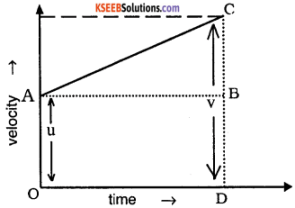Distance travelles by the particle is given by,
s = area under v-t graph
= area of the trapezium OACD
= 1/2 (OA+CD) × OD
But OA = u, CD = v and OD = t
∴ s = 1/2 (u + v) t or 2s = (u+v) t ………(1)
But, a = slope of the graph = $$\frac{v-u}{t}$$
∴ t = $$\frac{v-u}{a}$$
On substituting in (1), 2s = $$(u+v)\left(\frac{v-u}{a}\right)$$
i.e., v² – u² = 2as
or v² = u² + 2as.Question 7.
A particle is moving with uniform acceleration covers a distance of 45 m in 6th second and 75 m in 12th second during its motion. Calculate the displacement of the particle after 20s?
S6 = 45m, S12=75m
using Sn = u + a (n – 1/2 )
i.e., S6  = u + a or 45 = u + 5.5a ……… (1)
S12= u + a(12 – 1/2) or 75 = u + a(11.5) …….. (2)
Eq(2) – (1) gives 6 a = 30
Or a = 30/6 = 5m/s²
From (1), 45 = u + 5 × 5.5
u = 17.5m/s.
Distance travelled in t = 20s,
S = ut+ 1/2at2
= 17.5 × 20 + 1/2 × 5 × 20²
=1350m.

Question 8.
Draw a velocity-time graph of uniformly accelerated motion in one dimension. From the velocity-time graph of uniformly accelerated motion deduce the equations of motion in distance and time.
A graph drawn by taking time along the x-axis and velocity along the y-axis is called a velocity-time graph. Consider a particle moving along a straight line with a constant acceleration ‘a’. Let u be its velocity at time t = 0 and v be the velocity after t seconds. The velocity-time graph AC of this particle is shown in the figure.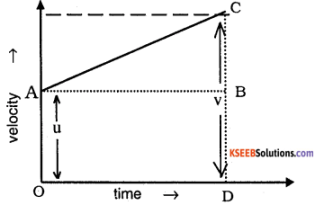Distance travelled by the particle in t seconds is given by,
s = area under v-t graph
= area of the triangle ABC + area of the rectangle ABDO
= 1/2 BC × AB + OA × OD From the graph,
BC = CD – BD = v – u,
AB = OD = t and OA = u
∴ s=1/2 ( v-u) t + ut …….. (1)
But, a = Slope of the graph = $$\frac{v-u}{t}$$
∴ v – u = at
On substituting in (1) we have,
s = 1/2 at.t + ut
s = ut + 1/2 at².

Question 9.
Derive an equation for the distance covered by a uniformly accelerated body in the nth second of its motion. A body travels half its total path in the last second of its fall from rest. Calculate the time of its fall.
Consider a particle moving with a uniform acceleration ‘a’.
Let u be the initial velocity of the particle.
Distance travelled during nth second = Distance travelled in ‘n’ seconds – Distance travelled in (n-1) seconds
sn = un + 1/2 an² – [ u(n – 1) + 1/2 a(n – 1)²]
= un + 1/2 an² – [ un – u + 1/2 a(n² – 2n + 1)]
= un + 1/2 an² – [ un – u + 1/2 an² – an + 1/2 a]
= un + 1/2 an² – un + u – 1/2 an² + an – 1/2 a
= u + an – 1/2 a
sn =u + a(n – 1/2)
sn = 4 + a (n – 1/2)
Given u = 0, a = g
s = 1/2 g t² = 1/2 g n²
By the given condition 1/2 s = sn
⇒ $$\frac{\mathrm{g} n^{2}}{4}$$ = g(n – 1/2)
⇒ n² – 4n + 2 = 0
⇒ n = $$\frac{4 \pm \sqrt{16-8}}{2}$$
⇒ n = 2 ± $$\sqrt{2}$$
∴ Time of the body’s fall = (2 ± $$\sqrt{2}$$) s

1st PUC Physics Motion in a Straight Line Numerical Problems Questions and Answers

Question 1.
A man runs from his home to office at a speed of 2 ms-1 on a straight road and returns back to home at a speed of 4 ms-1. Find

1. Average speed
2. average velocity.

Solution:
1. To find the average speed. Let ‘s’ is the distance between the home and the office.
∴ Time taken to reach the office
t1 $$\frac{\text { distance }}{\text { velocity }}$$ = $$\frac{s}{2}$$
Similarly, time taken to walk back to the home from the office is t2 = $$\frac{s}{4}$$
∴ Total time taken = t1 + 2 = $$\frac{s}{2}$$ + $$\frac{s}{4}$$ = $$\frac{3 s}{4}$$
Total distance moved = s + s = 2s
Hence. the average speed $$=\frac{\text { toal distance moved }}{\text { total time taken }}$$= 2.67 ms-1
2. To find the average velocity Since the man comes back to home, the final and initial position is same.
∴ Total displacement is zero.
Hence average velocity
$$=\frac{\text { toal displacement }}{\text { total time }}$$ = 0

Question 2.
A car moving along a straight high-way with a speed of 35 m/s is brought to stop within a distance of 200 m. What Is the retardation, and how long does it take for the car to stop? Solution:
The initial speed of the car, u = 35 ms-1
The final speed of the car, v = 0
Let a be the retardation of the car.
Then using the relation,
v² = u² + 2as, we have 0 = (35)² + 2a × 200
a = – $$\frac{35 \times 35}{2 \times 200}$$ = – 3.06 ms-2
Let t be the time taken by the car to come to stop. Then,
v = u + at ;
0 = 35 – 3.06 × t or
t = $$\frac{35}{3.06}$$ = 11.43 s.

Question 3.
A car starts from rest and accelerates from rest uniformly for 10 s to a velocity of 36 kmhr-1. It then runs at a constant velocity and is finally brought to rest in 50 m with uniform retardation. If the total distance covered by the car is 500 m, find

1. acceleration and
2. retardation.

Solution:
1. Initial velocity of the car u = 0;
Final velocity v = 36 kmhr-1
= $$\frac{36 \times 1000}{3600}$$ ms-1
= 10 ms-1
time taken t=10 s
∴ acceleration a = $$\frac{\mathrm{v}-\mathrm{u}}{\mathrm{t}}$$ = $$\frac{\mathrm{10}-\mathrm{0}}{\mathrm{10}}$$ 1ms-2

2. To find the retardation
During the motion with retardation, Initial velocity of the car u = 10 ms-1
Final velocity v = 0, distance travelled, s = 50 m
Using the relation v² = u² + 2as,
0 = 10² – 2a × 50
a = $$-\frac{100}{2 \times 50}$$ = -1 ms-2

Question 4.
A body travelling with a uniform acceleration travels a distance of 100m in the first 5 second and 200m in the next 5 second, calculate the initial velocity and acceleration of the body.
Solution:
If s1 and s2 are the distances travelled by a body in two successive intervals of t seconds, then the acceleration is given
by, a = $$\frac{s_{2}-s_{1}}{t^{2}}=\frac{200-100}{5^{2}}=\frac{100}{25}$$ = 4ms-2
we have, s = ut + 1/2 at²
Here, s = s1 = 100m, a = 4ms-2, t = 5 s
100 = u × 5 + 1/2 × 4 × 25 OR
5u = 50 ∴u= 10ms-1.Question 5.
A body moving with uniform acceleration along a straight line covers 60m in the 5th second and 80m in the 8th second of its motion. Calculate the initial velocity and uniform acceleration.
Solution:
The distance travelled during the nth second is given by,
sn = u + a/2(2n – 1), n = 5 and sn = 60
∴ 60 = u+ a/2 (2 × 5 – 1)
60 = u + 9a/2 ………… (1)
Similarly, for n = 8, sn =80
∴ 80 = u+ a/2 (2 × 8 – 1)
80 = u + 15a/2 …………….. (2)
(2) – (1), gives
20 = 6a/2 = 3a
∴ a = 20/3 ms-2
Using this in equation (1), we get
60 = u + $$\frac{9 \times 20}{2 \times 3}$$
u = 60 – 30 = 30 ms-1.

Question 6.
A stone is thrown vertically upwards with an initial velocity of 19.6 m-1. After how long will the stone strike the ground? Take g = 9.8 ms-2.
Solution:
Initial velocity u = +19.6 ms-1. The stone after reaching the highest point comes back to the initial point
∴ The displacement = 0.
a = g = – 9.8 ms-2
Let t be the time taken to reach the ground
From s = ut + 1/2at², we have
0=19.6t – 1/2 × 9.8 × t²
4.9t² – 19.6t = 0
Dividing throughout by 4.9t, we have t = 4s. Hence, the stone reaches the ground after 4s.Question 7.
A stone is thrown vertically upwards with a velocity of 10ms-1 from the top of a tower 40m tall and it finally falls to the ground,

1. Find the time taken by the stone to reach the ground
2. After how long will it pass through the point of projection
3. Calculate the velocity when it strikes the ground. Take g = 10 ms-2

Solution:
Let the stone be thrown upward with a velocity u = 10ms-1 from the tower AB of height 40 m. Let C be the highest point reached.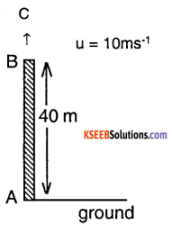1. Let t be the total time taken by the stone to move from B to C and back to the ground.
∴ The displacement = BA = – 40 m (directed downward since displacement is measured from the initial to the final position)
a = -10 ms-2.
From s = ut + 1/2at², we have
– 40= 10 × t – $$\frac{10 t^{2}}{2}$$
– 40 = 10t – 5t²
– 8 = 2t -t²
t² – 2t – 8 = 0
(t – 4) (t + 2) = 0.
OR
t = + 4s or – 2s
As time cannot be negative total time taken to reach the ground = 4s.

2. For the motion from B to C and back to B,
displacement = 0
u = 10ms-1, a = g = -10ms-2
Let t1 be the time taken.
From s = ut + 1/2at², we have
0= 10t1 – $$\frac{10 t_{1}^{2}}{2}$$ or 101² – 5t1² = 0
∴ t1 = 2s.
Hence, the stone reaches the point of projection after 2 seconds.

3. Let ‘v’ be the velocity of the stone when it strikes the ground. For the motion from B to C and back to A, we have,
u = 10 ms-1, a = g = -10 ms-2, t = 4s
From, v = u + at, we have
v = 10 – 10 × 4 = – 30 ms-1.

Question 8.
A stone is projected vertically upwards from the ground with a velocity of 49 ms-1. At the same time, another stone is dropped from a height 98m to fall freely along the same path as the first. Find where and when the two stones meet each other.
Solution: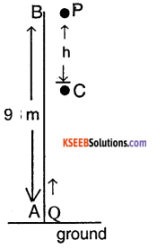Let P be the stone dropped from B at a height 98m from the ground A. Q is the other stone thrown up vertically upwards with a velocity 49ms-1 at the same instant. Let the two stones meet at C after a time t seconds.
For the stone P, we have
u = 0, a = + g, s = + h (=BC), t = t
From s = ut + 1/2 at², we have,
+h = 0 × t + 1/2 gt² or h = 1/2 gt² ………. (1)
For the stone Q,
u = 49ms-1,
a = -g,
s = +AC = +(98 – h),
(98 – h) = 49t – 1/2 gt²
Using eqn. (1) in (2), we have,
(98 – h) = 49t – h or 49t =98
∴ t = 2s.
Using this in (1), we have
h = BC = 1/2 × 9.8 × 4 = 19.6 m
∴ AC =96 – 19.6 = 76.4 m
∴ Two stones meet at a height of 76.4 m from the ground, after 2 seconds.

Question 9.
A train is moving southwards with a speed of 30ms-1. A man is running on the roof of the train with speed of 5ms-1 with respect to the train. Find the velocity of the man as observed by the person on the ground if the man is running

1. southwards
2. northwards.

Solution:
If vA and vB are the velocity of the two bodies moving along the same direction with respect to the ground, relative velocity of A with respect to B is given by,
VAB = VA – VB …………. (1)
Here, the velocity of the man with respect to the train,
vAB = 5ms-1.
velocity of the train with respect to the ground is,
vB = 30 ms-1
∴ velocity of the man with respect to the ground,
vA = vB + vAB = 30 + 5 = 35 ms-1 if the man moves southwards.
On the other hand, if the man moves northwards,
vAB = – 5 ms-1
∴ VA = VB – VAB
= 25 ms-1.Question 10.
A police van moving on a highway with a speed of 10ms-1 fires a bullet at a smuggler’s car speeding away in the same direction with a speed of 30ms-1. If the muzzle velocity of the bullet is 140ms-1, with what speed the bullet will hit the smuggler’s car?
Solution:
Speed of the police car vp = 10 ms-1.
Muzzle velocity of the bullet vM=140 ms-1
Net velocity of the bullet,
vA = vp + vM = 10 + 140 = 150 ms-1
(∵ gun is mounted on the van)
speed of the smuggler’s car vB = 30 ms-1
∴ Velocity of the bullet relative to smuggler’s car,
vAB = VA – VB
= 150 – 30 = 120 ms-1.

Question 11.
Two trains A and B are moving on two parallel tracks with a uniform speed of 20ms-1 in the same direction with A ahead of B. The driver of B decides to overtake A and accelerates by 1 ms-2. After 50 seconds, they are moving together, find the original distance of separation.
Solution:
Initially, both A and B are moving with the same velocity in the same direction,
∴ The initial relative velocity .
uAB = uA – uB = 0
when t = 50 s,
relative acceleration = aAB = 1 ms-2
If s is the distance moved, then
From s = ut + 1/2at²
S = uAB × t + 1/2 aAB
S = 0 × t + 1/2 × 1 × 50²
= 2500/2 = 1250 m.
Hence, the Initial distance of separation is 1250m.

Question 12.
A body is starting from rest and is subjected to a uniform acceleration of 10ms-2 determine.

1. Velocity of the body at the end of 5s
2. Displacement of the body In the first 3s
3. velocity of the body after a displacement of 20m.
4. Displacement of the body In the 4th second of its motion (Tumkur 05)

Solutions:
1. Initial velocity, u = 0
acceleration, a = 10ms-2
time, t = 5s,
v=?
From, the Equation, v = u + at we have,
v= 0 + 10 × 5
v= 50 ms-1

2. Initial velocity, u = 0
acceleration a = 10 ms-2
time, t = 36s
Distance travelled, s =?
From the equation s = ut+ 1/2 at² we have
= 0 + 1/2 × 10 × 9
s = 45 m

3. Initial velocity u= 0,
acceleration a = 10ms-2
Displacement s = 20m
velocity v=?
From the equation v²= u² + 2as ,
= 0² + 2 × 10 × 20
= 400
v = $$\sqrt{400}$$ = 20ms-1

4. Initial velocity u=0
Acceleration a = 10ms-1
n = 4 sec
Displacement S4 = ?
From the Equation, Sn = u+ a/2 (2n-1)
S4=0 + 10/2 (2 × 4 – 1) = 0 + 5(7)
S4 = 35m.Question 13.
A stone dropped from the top of a building travels 24.5 m in the last second of its fall. Find the height of the tower, (g = 9.8 m/s2)
Distance travelled in the last second of its fall is Sn = 24.5m
Also, Sn = ut + a (n – 1/2)
But u = 0, a = g
∴ Sn = 0 + g (n – 1/2)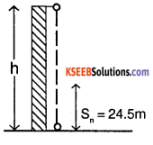24.5 = 9.8n – 4.9
n =$$\frac{24.5+4.9}{9.8}$$ = 3 seconds.
we know that S = ut + 1/2 at²
∴ Height of the tower;
h = 0 × 3+ 1/2 × 9.8(3)²
(∵ s = h, u = 0, a = g = 9.8m/s)
∴ h = 0 + 4.9(3)²
= 44.1m.

Question 14.
A body moving along a straight line with uniform acceleration covers 23m and 35m respectively In the 5th and 8th second of its motion calculate the distance travelled by the body in

1. 10th second
2. complete 10 second and what is its velocity at the end of 6 seconds.

S5 = 23m, S8 = 35m
W.k.that sn = u + a(n – 1/2)
i.e., S5 = u + a(5 – 1/2) or 23 = u + 4.5 a….(1)
S8 = u + a(8 – 1/2) or 35 = u + a(7.5)……….(2)
Eq(2) – (1) gives 3a = 12
or a = 12/3 = 4 m/s²
From (1), 23 = u + 4.5 × 4
u = 5 m/s
1. Distance traveled in 10th second
S10=u + a(n – 1/2) = 5 + 4(10 – 1/2) = 43m

2. Distance traveled in 10s is
S= ut + 1/2at² = 5 × 10 + 1/2 × 4 × 10² = 250m
Velocity at the end of 6 seconds is v = u + at = 5 + 4 × 6 =29 m/s.

Question 15.
A point object is thrown vertically upwards at such a speed that it returns the thrown after 6 seconds. With what speed was it thrown up and how high did it rise? Plot speed-time graph for the object and use it to find the distance travelled by it in the last second of its journey.
Time of rise t1 = time of fall = t2
t = 6 s
v = u – g t (upward direction is chosen as positive)
v = – u
– u=u-gt
⇒ – 2u = – 9.8 × 6
⇒ u = 29.4 ms-1
v² = u² – 2 gh
At the top, v = 0
⇒ h = $$\frac{u^{2}}{2 g}$$
= $$\frac{(29.4)^{2}}{2 \times 9.8}$$
h = 44.1 m
Initial speed = 29.4 ms-1
Height the object attained = 44.1 mDistance covered in last second = area under the speed-time graph.
= 1/2 × (6 – 5) × (19.6 + 29.4)
= 24.5 m

Question 16.
A race car is moving on a straight road with a speed of 180 km h-1. If the driver stops the car in 25 s by applying the brakes, calculate the distance covered by the car during the time brakes are applied. Assume acceleration of the car is uniform throughout the retarding motion.
Initial speed μ = 180 km h-1
μ = 50 ms-1
t = 25 s
v = u – at
v = 0
∴ 0 = 50 – a × 25
⇒ a = 2 ms-2
v² = u² – 2as
⇒ s = μ²/2 a
$$\frac{50^{2}}{2 \times 2}$$
∴ Stopping distance = 625 m

Question 17.
A body covers 12 m in the 2nd second and 20 m in the 4th second. Find what distance the body will cover in the 4 seconds after the 5th second.
sn= u + a/2 (2n -1)
s2 = 12
⇒ 12 = u+ a/2 (2 × 2 – 1)
⇒ 12 = u + 3 a/2 ………. (1)
s4 = 20
⇒ 20 = u+ a/2 (2 × 4 – 1)
⇒ 20 = u + 7a/2 ………. (2)
Subtract equation (1) from (2)
s = 4a/2
⇒ a = 4 ms-2
12 = u + 3/2 × 4
⇒ u = 6 ms-1
Distance covered in the 4 seconds after 5th second = S9 – S5
= u (9) + 1/2 a (9)² – [u(5) + 1/2 a (5)² ]
= 4u + a/2 (81 – 25)
= 4 × 6 + 4/2 × 56 = 136 m.Question 18.
A ball is thrown vertically upwards with a velocity of 20 ms-1 from the top of a building of height 25 m from the ground.

1. How high will the ball reach?
2. How long will the ball tale to reach the ground ? (g = 10 ms-2)

u = 20 ms-1
h = 25 m
g = 10ms-2
1. v² = u² – 2gh1
At the top, v = 0
⇒ h1 = $$\frac{\mathrm{u}^{2}}{2 \mathrm{g}}$$
= $$\frac{20^{2}}{2 \times 10}$$
= 20 m
∴ Height the ball reaches = 20 + 25 = 45 m from the ground.
2. – h = u t – 1/2 g t²
– 25 = 20 t – 1/2 × 10 t²
⇒ 5 t² – 20 t – 25 = 0
⇒ t² – 4 t – 5 = 0
⇒ (t – 5 ) (t + 1 ) = 0
= t = 5, -1
∴ t = 5 s (Since t cannot be negative) Total time to reach the ground = 5 s

1st PUC Physics Motion in a Straight Line Hard Questions and Answers

Question 1.
The speed of a train increases at a constant rate from zero to v and then remains constant for an interval, and finally decreases to zero at a constant rate p. If L be the total distance covered prove that the total time taken is $$\frac{L}{v}+\frac{v}{2}\left(\frac{1}{\infty}+\frac{1}{\beta}\right)$$
During time t1, the train accelerates uniformly from 0 to V.
v = α t1
Distance covered d1 = 1/2 α t1²
= 1/2 (∝ t1) t1
d1 = 1/2 v t1
⇒ $$t_{1}=\frac{2 d_{1}}{v} \alpha$$
During time t2, the train travels at a uniform speed of v
Distance covered d2 = vt2
⇒ t2 = $$\frac{\mathrm{d}_{2}}{\mathrm{v}}$$
During time t3, the train decelerates uniformly from v to 0.
0 = v – β t3
⇒ v = β t3
Distance covered d3 = vt3 – 1/2 β t3²
= vt3 – 1/2 (β t3) t3
d3 = vt3 – 1/2 v t3
⇒ d3 = 1/2 v t3
⇒ t3 = $$\frac{2 \mathrm{d}_{3}}{\mathrm{v}}$$
Total time T = t1 + t2 + t3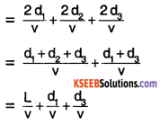Using v² = u² + 2as
d1 = $$\frac{v^{2}}{2 \alpha}$$ and d3 = $$\frac{v^{2}}{2 \beta}$$
∴ T = $$\frac{L}{v}+\frac{v}{2 \alpha}+\frac{v}{2 \beta}$$
T = $$\frac{L}{v}+\frac{v}{2}\left(\frac{1}{\alpha}+\frac{1}{\beta}\right)$$
Hence proved.

Question 2.
In a car race, car A takes ‘t’ seconds less than car B and passes the finish line with a velocity V’ more than that of car B. Of the cars start from rest and travel with constant acceleration a1 and a2 respectively, show that
V = $$t \sqrt{a_{1} a_{2}}$$.
Let car A take tA seconds to finish the race.
Car B takes tB seconds to finish the race. Let the final velocities of the cars be vA and vB
By the given conditions,
tB – tA = t
vA = a1 tA
vB = a2 tB
vA – vB = v
Since the distance d covered is the same,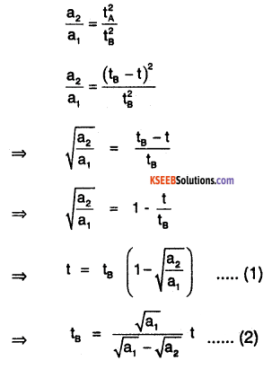Now, vA = a1 tA
⇒ vB + v = a1 (tB – t)
⇒ a2 tB + v = a1 (tB – t)
⇒ v = (a1 – a2) tB – a1 t
Substitute for t from (1),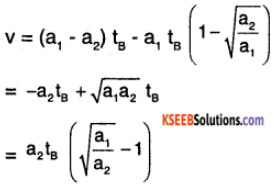Substitute for tB from (2),Hence proved.

Question 3.
An object moves with a deceleration $$\alpha \sqrt{\mathbf{v}}$$ in a straight line (a is a constant) At time t = 0, the velocity is v0. What is the distance it traverses before coming to rest? What will be the total time taken?
Deceleration = $$\alpha \sqrt{\mathbf{v}}$$
⇒ Acceleration a = – $$\alpha \sqrt{\mathbf{v}}$$
$$\frac{\mathrm{d} \mathrm{v}}{\mathrm{dt}}$$ = – αv1/2
⇒ $$\frac{\mathrm{d} \mathrm{v}}{\mathrm{v}^{1 / 2}}$$ = – αdt
Integrating,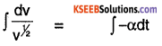2v1/2 = – αt + c
At t = 0, v = v0
⇒ 2v01/2 = c
∴ 2v1/2 = – αt + 2v01/2 …………… (1)
When t = T, v = 0
∴ 2(0)1/2 = – αT + 2v01/2
⇒ T = $$\frac{2 \sqrt{v_{0}}}{\alpha}$$
∴ Total time taken = $$\frac{2 \sqrt{v_{0}}}{\alpha}$$
From (1), we geterror: Content is protected !!